# Discovering the Sum of Reciprocal Powers

Having found the formula for sum of powers, I was curious to see how the reciprocals of powers behave in the same situation.

Let's start with reciprocal of powers of 2. When we are dealing with reciprocals, note that the reciprocals of powers form a decreasing sequence. In other words,Which means, if we try to do it like before, adding up lower powers to get the next one, it's not going to work. Because, the the next reciprocal is LESS than the sum of reciprocal of lower powers.
Eg: If we add up 1/2 + 1/2^2 as below,

1/2 + 1/2^2 = 1/2 + 1/4 = 3/4 , it turns out to be more than the next reciprocal power, that is 1/8.

So we need to try something else. In the previous case of adding the powers, we had an expression + 1 at the end of the product (n-1)(n^k + n^k-1 + ... + n^0). So this time, lets SUBTRACT FROM 1.

Let's try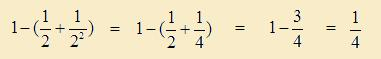The end result is 1/4. But we need 1/2^3, which is 1/8. Before we try another one, we make an important observation : 1/8, the number we seek is 1/2 times 1/4.

Let's try with the next power of 2.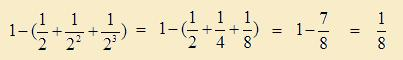Again, the number we seek, 1/16, is 1/2 times 1/8.
Which means, all, the while, we should have multipled the sum by 1/2.
Let's apply this correction and find out if our sums come out right.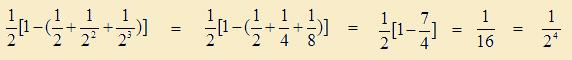Hooray! It works.

Let's see what happens with reciprocal powers of 3. This time, taking cue from the previous discovery, we will multiply the expression in the square brackets by 1/3.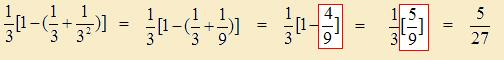and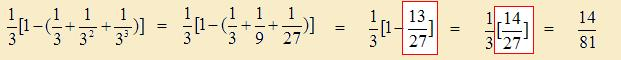Uh-oh. This does not seem to work with 3. What's wrong? We do notice that the result we get has the correct denominator that we are looking for (27 & 81). Probably, we are close to the secret. Just that we should have had 1 in the numerator instead of 5 & 14.
How can we correct this?

Looking at the previous article, we see that we had a term (n-1) appearing in the formula. But it does not appear here. The world of reciprocals must be similar to the world of non-reciprocals. We are probably missing this (n-1) somewhere.

Let's go back to our calculation for powers of 3 and see what adjustment we can make to get the result we want. Note that we have highlighted the term 4/9 in the first result of powers of 3.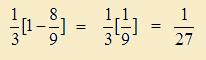Exactly what we want!
Similarly, if we had 26/27, instead of the highlighted 13/27 in the second example, we would have had,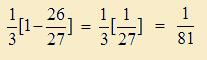Now we are getting somewhere. The numerator of the highlighted term in both cases, should have been double! Which means, this is where we have been missing the term (n-1), which is 3 - 1 = 2, in our case.
So, the correct formula should have beenWhich is perfect!
Let's check for 4, before we write our general formula. If we are right, then in case of 4, the multiplier similar to the one highlighted in equations above, will be 4 - 1 = 3. Let's find out.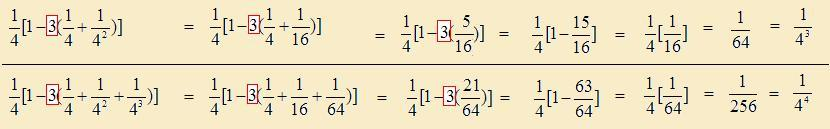That works! Based on our observations so far, we are now ready to write down the general formulaNow, let's formally prove our formula for the general case.
Property :    For natural numbers n >= 1,Proof :
The proof is trivial, like in the previous case.Quick Comment : * Name Email ( Your email id is safe with us! ) * Comments * Type the number of characters in the word "HUMAN"